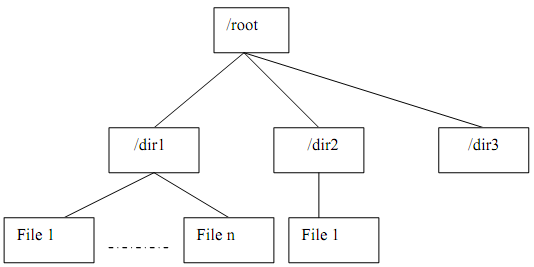## Post order traversal, Data Structure & Algorithms

Assignment Help:

Post order traversal:

The children of node are visited before the node itself; the root is visited last. Each node is visited after its descendents are visited.

Algorithm for postorder traversal is following:

1. Traverse left sub-tree in post order

2. Traverse right sub-tree in post order

3. visit root node.

Determining the space occupied through files & directories in a file system need a postorder traversal as the space occupied through directory needs calculation of space needed by all files in the directory (children in tree structure)Figure:  Calculation of space occupied by a file system: A post order traversal

Since each of the nodes is traversed only once, the time complexity of post order traversal is T(n) = O(n), where n refer to number of nodes in the tree.

#### File organisation, File organisation might be described as a method of stor...

File organisation might be described as a method of storing records in file. Also, the subsequent implications approaching these records can be accessed. Given are the factors invo

#### Method to add an element in circular queue, Q. Let us consider a queue is h...

Q. Let us consider a queue is housed in an array in circular fashion or trend. It is required to add new items to the queue. Write down a method ENQ to achieve this also check whet

#### Explain insertion procedure into a b-tree, Ans: I nsertion into the B...

Ans: I nsertion into the B-tree: 1.  First search is made for the place where the new record must be positioned. As soon as the keys are inserted, they are sorted into th

#### Breadth-first search , 1. Apply the variant Breadth-First Search algorithm ...

1. Apply the variant Breadth-First Search algorithm as shown in Figure 2 to the attached graph. This variant is used for computing the shortest distance to each vertex from the sta

#### Explain the abstract data type assertions, Explain the Abstract data type a...

Explain the Abstract data type assertions Generally, ADT assertions translate into assertions about the data types which implement ADTs, which helps insure that our ADT impleme

#### Linked lists - implementation, The Linked list is a chain of structures whe...

The Linked list is a chain of structures wherein each structure contains data in addition to pointer, which stores the address (link) of the next logical structure in the list.

#### Array and two-dimensional array, Q. Describe the term array.  How do we rep...

Q. Describe the term array.  How do we represent two-dimensional arrays in memory?  Explain how we calculate the address of an element in a two dimensional array.

#### Explain best - fit method, Best - Fit Method: - This method obtains the sma...

Best - Fit Method: - This method obtains the smallest free block whose  size is greater than or equal to get such a block by traversing the whole free list follows.

#### Financial index data analysis, need c++ algorithmic software program to der...

need c++ algorithmic software program to derive one numerical outcome from 10 levels of variables with 135 combinations cross computed

#### Data mining assignment, The assignment aims at consolidating your knowledge...

The assignment aims at consolidating your knowledge on data mining techniques and developing practical skills through solving a problem of transcription factor binding sites recogn